# Determining a Missing Data Value Given the Mean

In this section we will be looking at examples where we have been given the average or mean but we are missing one of the values from the set that has that mean.

This might sound kind of confusing, so let's get right to some examples.

Example #1:

Sunil wants to earn an 88% in her Chemistry class. She has one quiz left to take.

On the first 5 quizzes, she received a 74%, 92%, 83%, 98%, 100%. What must she score on the 5th quiz to get the 88% she wants?

Step 1: Recall the steps for determining the mean.

We would add up all of the scores and divide by how many scores there are.

Step 2: Set up the problem as if you were determining the mean. We can use an x to represent the quiz grade that we don't know.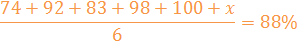Step 3: Now we are ready to solve.

Start by multiplying both sides by 6.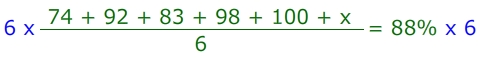74 + 92 + 83 + 98 + 100 + x = 528

Next, we will add all the values together on the left side.Finally, we will subtract the 447 from the 528 points we need to earn total to determine the score of the 6th quiz.Let's check out another example.

Example #2:

Frank wants to have a bowling average of 215.

The first 7 games he scored 159, 182, 225, 240, 198, 200, 230. What must he score in the 8th game to have an average of 215?

Step 1: Set up the problem using the method for determining the mean.Step 2: Multiply both sides by 8 to eliminate the fractions.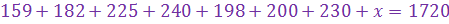Step 3: Add up the scores on the left side of the equation.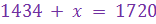Step 4: Subtract this value from the 1720.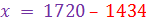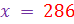Frank would have to score a 286 in order to have a bowling average of 215.

Let's Review:

If you are given a mean and asked for one of the data values, you can still use the basic method for determining the mean, but work backwards. Generally we add up all the values and then divide by the number of values. In this case, working backwards, we multiply by the number of values (instead of dividing) and then subtract (instead of adding). You should be left with a data value from the set.